×資料管理 統計分析 相關資源 巨人肩膀 語法索引 關於作者

## 簡介

• lm() -線性回歸
• confint() -計算信賴區間
• coefficient() -計算回歸模型的係數
• fitted() -根據回歸模型計算應變數
• residuals() -殘差
• layout() -設定R輸出圖形的格式
• plot() -繪製散布圖
• hatvalues() -計算槓桿值
• cooks.distance() -計算庫克距離
• abline() -繪製直線
• line() -繪製線條
• text() -加上文字
• I() -指出(Indicate)物件的處理方法
• poly() -多項式回歸
• scatterplot3d() -*3D散布圖
• vif() -計算變異數膨脹因子
• probe_interaction() -繪製交互作用圖
• sim_slopes() -估計交互作用斜率
• center() -將變數平均中心化

$y=\alpha x + \beta + \varepsilon, \varepsilon \sim N(0,\sigma^2)$

$\alpha=\frac{n\displaystyle\sum_{j=1}^{n}{x_{j}}{y_{j}}-\displaystyle\sum_{j=1}^{n}{x_{j}}\displaystyle\sum_{j=1}^{n}{y_{j}}}{n\displaystyle\sum_{j=1}^{n}{x_{j}}^2-\left(\displaystyle\sum_{j=1}^{n}{x_{j}}\right)^2}, \beta=\frac{1}{n}\left(\displaystyle\sum_{j=1}^{n}y_{j}-\alpha\displaystyle\sum_{j=1}^{n}x_{j}\right)$

## 簡單線性回歸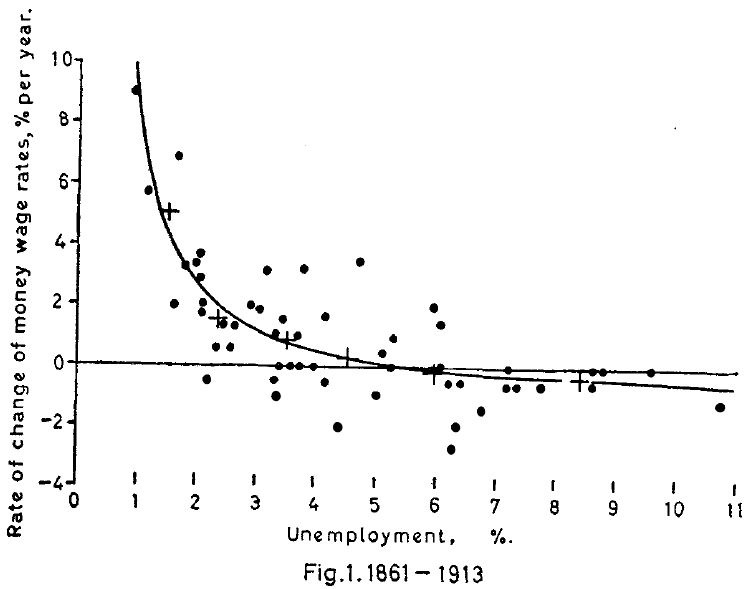### 回歸模型

 > phillips<-read.csv("c:/Users/USER/downloads/phillips_curve.csv", header=T, sep=",") > names(phillips) #顯示變數名稱  "year" "wage" "unemployment" "cpi"  "growth" > model<-lm(wage~unemployment, data=phillips) #建立工資率與失業率的回歸模型 > summary(model) Call: lm(formula = wage ~ unemployment, data = phillips) Residuals: Min 1Q Median 3Q Max -2.2673 -1.6501 -0.5478 1.2494 6.7699 Coefficients: Estimate Std. Error t value Pr(>|t|) (Intercept) 11.7449 1.3128 8.946 1.46e-09 *** unemployment -2.4400 0.3533 -6.906 2.02e-07 *** --- Signif. codes: 0 ‘***’ 0.001 ‘**’ 0.01 ‘*’ 0.05 ‘.’ 0.1 ‘ ’ 1 Residual standard error: 2.339 on 27 degrees of freedom (1 observation deleted due to missingness) Multiple R-squared: 0.6385, Adjusted R-squared: 0.6252 F-statistic: 47.7 on 1 and 27 DF, p-value: 2.02e-07 

 > confint(model, level=0.95) #信賴區間 > coefficients(model) #模型的係數與截距 > fitted(model) #依據模型所計算出來的y值 > residuals(model) #模型的殘差 

 > plot(x=phillips$unemployment, y=phillips$wage, xlab="Unemployment, %", ylab="Rate of Change of Money Wage Rates, % per year") #繪製散布圖 > abline(lm(phillips$wage~phillips$unemployment)) #在散布圖上加上回歸線 > text(x=3, y=8, col="red", "y=-2.44x+11.75") #在散布圖上加上回歸方程式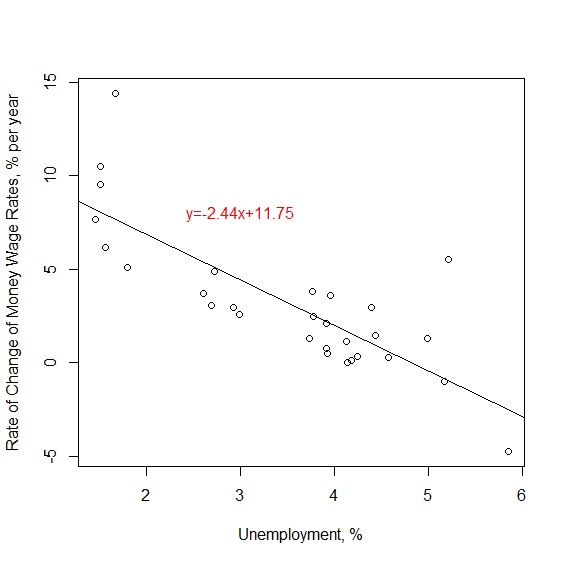### 判定係數$$R^2$$

 > anova(model) Analysis of Variance Table Response: wage Df Sum Sq Mean Sq F value Pr(>F) unemployment 1 261.03 261.033 47.698 2.02e-07 *** Residuals 27 147.76 5.473 --- Signif. codes: 0 ‘***’ 0.001 ‘**’ 0.01 ‘*’ 0.05 ‘.’ 0.1 ‘ ’ 1 

$$R^2=\frac{SSR}{SST}=\frac{261.03}{261.03+147.76}=0.6385$$

## 模型診斷

 > residuals(model) #計算殘差 > influence(model) #輸出回歸模型診斷數據 

### 圖形診斷

 > layout(matrix(c(1,2,3,4),ncol=2, nrow=2, byrow=TRUE)) #設定2x2的layout來呈現4張殘差圖 > plot(model) #呼叫4張殘差診斷圖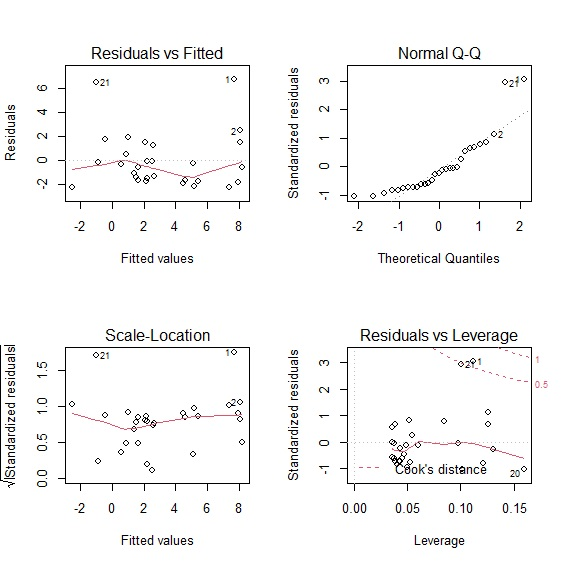### 槓桿值與庫克距離

 > library(car) #載入car套件 > layout(matrix(c(1), ncol=1, nrow=1)) #把圖形呈現的layout改回1x1 > influencePlot(model, main="Circle size is % of Cook's Distance") #繪製回歸模型診斷圖，並輸出離群值的槓桿值與庫克距離 StudRes Hat CookD 1 3.7339258 0.1114113 0.590828905 4 -0.2509052 0.1309457 0.004913307 20 -1.0468919 0.1597369 0.103806013 21 3.4950645 0.1006647 0.483014611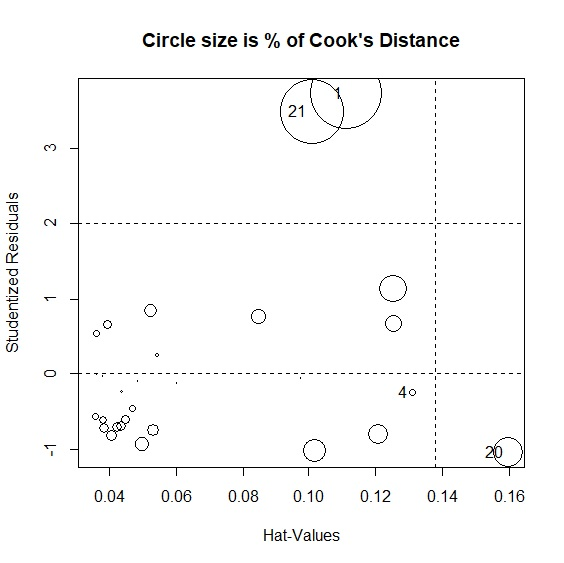> hatvalues(model) #計算每一個樣本的槓桿值 > cooks.distance(model) #計算每一個樣本的庫克距離 

### 殘差檢定

 > shapiro.test(model$residual) #殘差常態分配檢定 Shapiro-Wilk normality test data: model$residual W = 0.80777, p-value = 0.0001155 > durbinWatsonTest(model) #殘差常態自我相關(獨立性)檢定 lag Autocorrelation D-W Statistic p-value 1 0.2252316 1.227507 0.04 Alternative hypothesis: rho != 0 > ncvTest(model) #變異數同質性檢定 Non-constant Variance Score Test Variance formula: ~ fitted.values Chisquare = 0.3012812, Df = 1, p = 0.58308 

## 多元線性回歸 / 複回歸

 > MLR<-lm(wage~unemployment+growth, data=phillips) > summary(MLR) Call: lm(formula = wage ~ unemployment + growth, data = phillips) Residuals: Min 1Q Median 3Q Max -2.2662 -1.1801 -0.6977 1.2615 7.2979 Coefficients: Estimate Std. Error t value Pr(>|t|) (Intercept) 8.2170 1.8964 4.333 0.000195 *** unemployment -1.9822 0.3766 -5.264 1.68e-05 *** growth 0.4035 0.1671 2.415 0.023076 * --- Signif. codes: 0 ‘***’ 0.001 ‘**’ 0.01 ‘*’ 0.05 ‘.’ 0.1 ‘ ’ 1 Residual standard error: 2.155 on 26 degrees of freedom (1 observation deleted due to missingness) Multiple R-squared: 0.7048, Adjusted R-squared: 0.6821 F-statistic: 31.03 on 2 and 26 DF, p-value: 1.295e-07 

 > library(scatterplot3d) #載入scatterplot3d套件 > s3d<-scatterplot3d(phillips$unemployment, phillips$wage, phillips$growth, #繪製立體3D散步圖，並將結果指定給s3d + xlab="Unemployment", ylab="Change % of Wage Rate", zlab="Economic Growth", + main="Multiple Linear Regression(MLR)") s3d$plane3d(MLR, col="blue") #在s3d圖中繪製多元回歸模型平面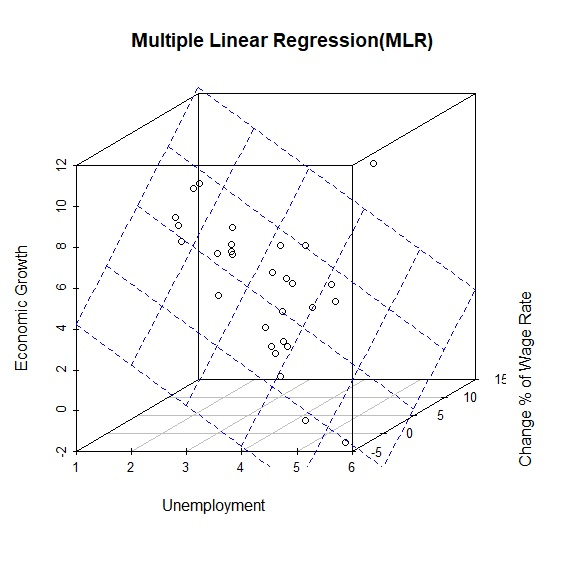### 共線性

 > cor(phillips, use="complete.obs") #排除遺漏值後進行相關分析 year wage unemployment cpi growth year 1.0000000 -0.6252511 0.7062332 -0.5913482 -0.5159854 wage -0.6252511 1.0000000 -0.7990892 0.6952941 0.6246005 unemployment 0.7062332 -0.7990892 1.0000000 -0.7609087 -0.5033919 cpi -0.5913482 0.6952941 -0.7609087 1.0000000 0.4705541 growth -0.5159854 0.6246005 -0.5033919 0.4705541 1.0000000 

 > vif(MLR) unemployment growth 1.339411 1.339411 

### 交互作用

$$y=\alpha+\beta_{1}x_{1}+\beta_{2}x_{2}+\beta_{3}x_{1}x_{2}+\epsilon$$

$$y=\alpha+\beta_{1}x_{1}+\beta_{2}x_{2}+\beta_{3}x_{1}x_{2}+\epsilon$$

$$=\alpha+\beta_{1}x_{1}+\beta_{3}x_{1}x_{2}+\beta_{1}x_{2}+\epsilon$$

$$=\alpha+(\beta_{1}+\beta_{3}x_{2})x_{1}+\beta_{2}x_{2}+\epsilon$$

$$=(\alpha+\beta_{2}x_{2})+(\beta_{1}+\beta_{3}x_{2})x_{1}+\epsilon$$

 > MLR_mod<-lm(wage~unemployment+growth+unemployment*growth, data=phillips) > summary(MLR_mod) Call: lm(formula = wage ~ unemployment + growth + I(unemployment * growth), data = phillips) Residuals: Min 1Q Median 3Q Max -2.2903 -1.1125 -0.4786 1.3615 7.0682 Coefficients: Estimate Std. Error t value Pr(>|t|) (Intercept) 10.60412 4.40133 2.409 0.0237 ** unemployment -2.51309 0.95974 -2.619 0.0148 * growth 0.03940 0.62732 0.063 0.9504 unemployment:growth 0.08066 0.13382 0.603 0.5521 --- Signif. codes: 0 ‘***’ 0.001 ‘**’ 0.01 ‘*’ 0.05 ‘.’ 0.1 ‘ ’ 1 Residual standard error: 2.181 on 25 degrees of freedom (1 observation deleted due to missingness) Multiple R-squared: 0.709, Adjusted R-squared: 0.6741 F-statistic: 20.3 on 3 and 25 DF, p-value: 6.986e-07 

$$y=10.60+0.04x_{2}+(-2.51+0.08x_{2})x_{1}$$

 > library(interactions) > probe_interaction(MLR_mod, pred=unemployment, modx=growth, plot.points=TRUE, interval=TRUE) JOHNSON-NEYMAN INTERVAL When growth is INSIDE the interval [-3.00, 11.56], the slope of unemployment is p < .05. Note: The range of observed values of growth is [-1.61, 10.25] SIMPLE SLOPES ANALYSIS Slope of unemployment when growth = 1.944998 (- 1 SD): Est. S.E. t val. p ------- ------ -------- ------ -2.36 0.73 -3.24 0.00 Slope of unemployment when growth = 4.765172 (Mean): Est. S.E. t val. p ------- ------ -------- ------ -2.13 0.45 -4.71 0.00 Slope of unemployment when growth = 7.585347 (+ 1 SD): Est. S.E. t val. p ------- ------ -------- ------ -1.90 0.40 -4.70 0.00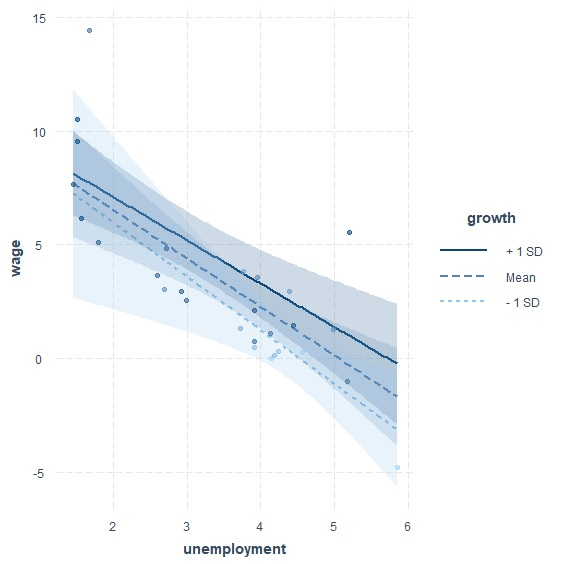> sim_slopes(MLR_mod, pred=unemployment, modx=growth, modxvals=c(0,5,10)) JOHNSON-NEYMAN INTERVAL When growth is INSIDE the interval [-3.00, 11.56], the slope of unemployment is p < .05. Note: The range of observed values of growth is [-1.61, 10.25] SIMPLE SLOPES ANALYSIS Slope of unemployment when growth = 0.00: Est. S.E. t val. p ------- ------ -------- ------ -2.51 0.96 -2.62 0.01 Slope of unemployment when growth = 5.00: Est. S.E. t val. p ------- ------ -------- ------ -2.11 0.44 -4.84 0.00 Slope of unemployment when growth = 10.00: Est. S.E. t val. p ------- ------ -------- ------ -1.71 0.60 -2.87 0.01 

$$y=10.60+0.04x_{2}+(-2.51+0.08x_{2})x_{1}$$

 > library(jtools) #載入jtools套件 > phillips_center<-center(phillips, binary.inputs="center") #將phillips的所有變數平均中心化 > MLR_mod2<-lm(wage~unemployment+growth+unemployment*growth, data=phillips_center) #用平均中心化的資料再跑一次回歸模型 > vif(MLR_mod) #平均中心化之前的模型共線性 unemployment growth unemployment:growth 8.48694 18.41673 14.10179 > vif(MLR_mod2) #平均中心化之後的模型共線性 unemployment growth unemployment:growth 1.883885 2.190252 1.710065 

$$y=\alpha+\beta_{1}x_{1}+\beta_{2}x_{2}+\beta_{3}x_{3}+\beta_{4}x_{1}x_{2}+\beta_{5}x_{1}x_{3}+\beta_{6}x_{2}x_{3}+\beta_{7}x_{1}x_{2}x_{3}+\epsilon$$

$$y=\alpha+\beta_{1}x_{1}+\beta_{3}x_{3}+\beta_{5}x_{1}x_{3}+\epsilon$$

$$y=\alpha+\beta_{1}x_{1}+\beta_{2}x_{2}+\beta_{4}x_{1}x_{2}+\epsilon$$